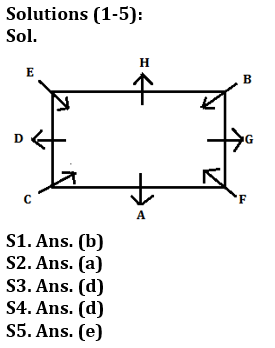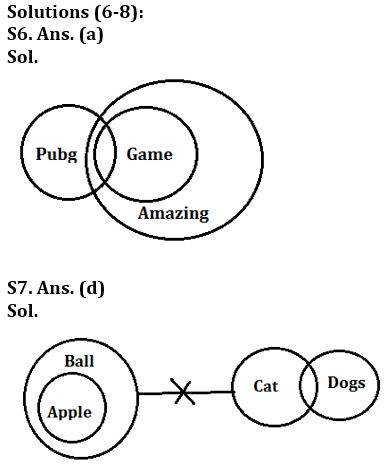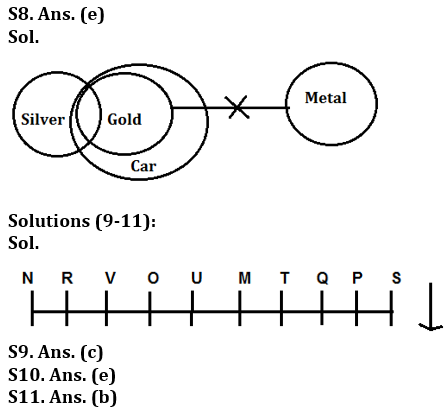Latest Banking jobs   »

# Reasoning Quiz For Bank of Baroda AO 2023-2nd March

Directions (1-5): Study the information carefully and answer the questions given below.
Eight persons i.e. A, B, C, D, E, F, G and H sit at square table. Four of them sit at corners and face inside. Remaining four sits at middle of the side and face outside. D sits second to the right of A. Two persons sit between D and F. G does not face D. H sits third to the right of F. B sits opposite to C. E sits second to the right of B.

Q1. Who among the following person sits second to the right of G?
(a) H
(b) A
(c) C
(d) E
(e) G

Q2. Who among the following person sits third to the right of C?
(a) G
(b) A
(c) F
(d) D
(e) H

Q3. How many persons sit between A and D when counted from left of A?
(a) One
(b) Two
(c) Three
(d) Five
(e) Four

Q4. Which of the following statement is true regarding E?
(a) E sits immediate right of B
(b) E sits second to the left of B
(c) None is true
(d) E sits at one of the corners
(e) E face outside

Q5. Four of the following five are alike in certain way based from a group, find the one that does not belong to that group?
(a) A-F
(b) B-H
(c) C-A
(d) D-E
(e) G-A

Direction (6-8): In each of the questions below some statements are given followed by two conclusions. You have to take the given statements to be true even if they seem to be at variance with commonly known facts. Read all the conclusions and then decide which of the given conclusions logically follows from the given statements disregarding commonly known facts.
(a) If only conclusion I follows.
(b) If only conclusion II follows.
(c) If either conclusion I or II follows.
(d) If neither conclusion I nor II follows.
(e) If both conclusions I and II follow.

Q6. Statements: Only a few Pubg are Game.
All Game are Amazing
Conclusion: I: All Game is Pubg is a possibility.
II: All Pubg are Amazing.

Q7. Statements: Only Ball is Apple.
No Ball is Cat.
Only a few Cat are Dogs
Conclusion: I: Some Apples can be Dogs
II: All Dogs can be Ball

Q8. Statements: Only a few Silver are Gold.
All Gold are Car.
No Gold is metal.
Conclusion: I: Some Car are not Metal.
II: Some metal can be Silver.

Directions (9-11): Study the following information carefully and answer the question given below-
There are ten people i.e. M, N, O, P, Q, R, S, T, U and V who have seated on a bench, faces towards South direction. M and T sits together but both of them do not sit at any extreme end. There a 4 people sit between Q and V. Only two people sits left to Q. O sits immediate right of U. P sits 2nd left of T and S is the neighbor of P. There is three people between U and N who does not sit at left end of the bench.

Q9. Who among the following sits 2nd left of Q?
(a) R
(b) M
(c) S
(d) N
(e) None of these

Q10. How many people sit between U and M?
(a) Four
(b) Three
(c) Two
(d) One
(e) No one

Q11. Who among the following sits at extreme right end?
(a) P
(b) N
(c) S
(d) T
(e) V

Q12. If all the letters in the word “REASONABLE” are arranged in alphabetical order from left to right in such a way that all vowels are arranged first followed by consonants, then how many letters are there in between O and S after the arrangement?
(a) Two
(b) One
(c) None
(d) Three
(e) Four

Q13. If in the number 639429687, 1 is added to each of the digit which is less than five and 1 is subtracted from each of the digit which is greater than five then how many digits are repeating in the number thus formed?
(a) Two
(b) One
(c) None
(d) Three
(e) Four

Q14. Which of the following elements should come in a place ‘?’ ?
DF8 HJ12 LN16 ?
(a) PR19
(b) PR18
(c) PR21
(d) PR22
(e) None of these

Q15. How many pairs of letters are there in the word ‘PERSPECTIVE’, each of which have as many letters between then in the word as they have between then in the English alphabet?
(a) Two
(b) One
(c) Four
(d) More than Four
(e) Three

SolutionsS12. Ans. (e)
Sol. After arrangement: AAEEOBLNRS

S13. Ans(a)

S14. Ans. (e)
Sol. PR20

S15. Ans. (d)
Sol. PR, PS, RS, PR, TV## FAQs

### What is the selection process of Bank of Baroda AO 2023?

The selection process of Bank of Baroda AO consists of Online exam and Interview

#### Congratulations!Union Budget 2023-24: Free PDF